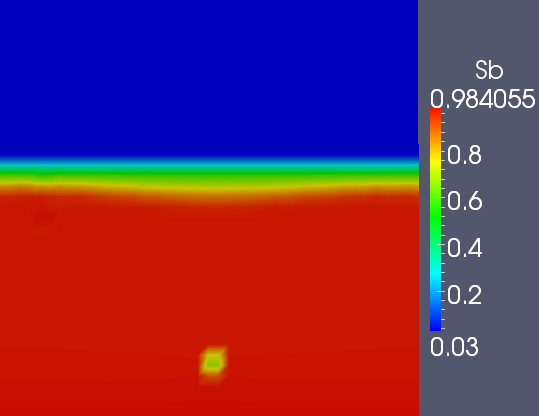# Simulation of different regimes (without capillary pressure)

The aim of this part is to highlight the existence of different regimes that depend on the source term ( flow rate in the well) , on the fluid and the ground properties.

We will launch different simulations for different rate flow values to detect those regimes.

To study the existence of different regime we will plot the saturation for different times and for different flow rates values.

The domain dimension for all the following simulations is 10m*10m.

# Oil production : low flow rate regime

We have a mesh 40*40 cells and the a well of 20cm*50cm.

In the following simulation when the oil saturation goes under the value 0.1 we stop the well production.

we had the following parameters :

phase a :

•  Viscosity $\mu_a=1.17\ kg/(m.s)$
• density $\rho_a=1.85 \ 10^{-5} kg/m^3$

phase b :

•  Viscosity $\mu_b=1 \ 10^{-1} kg/(m.s)$
• density $\rho_b=0.88 \ 10^3 Kg/m^3$

The initial flow rate qwell was fixed to :

•  $qwell=5 \ 10^{-5} m^3/s$

Moreover we consider that we have an isotropic domain and we fixed the permeability to :

•  $K=1 \ 10^{-9}m^2$

Here we can see the representation of the domain :

Well and domain representationThis the initial saturation that  has been taken :

Initial oil staurationSaturation in oil time=63500 sSaturation in oil time=203500 sAs the oil considered in those simulations is very viscous ( $\mu_b=1 \ 10^{-1} kg/(m.s)$ ) this decreases the mobility and it is not possible to pump with high value of the rate flow. Indeed pumping very viscous liquid with high rate flow results in a creation of a "hole in the domain" and the introduction of air in the well which disturb significantly the production. The rate flow adapted to this liquid/configuration should be around $qwell=1 \ 10^{-5} m^3/s$}.

Example of " hole" in the saturation created in the case of $qwell=5 10^{-5} \ m^3/s$ and $K=10^{-10} m^2$Indeed we can see on the previous figure that with very viscous fluid and very low permeability we cannot pump with very high flow rates.

# Water production : high flow rate regime

We have a mesh 120*120 cells and the a well of 50cm*200cm.

We took in this part of fluid that have properties similar to the water properties but less dense than water.

Here we can see the representation of the domain :

Well and domain representationThe initial saturation will have the form of this :

Initial saturation in waterWe implemented a first simulation with the following parameters

phase a :

• Viscosity $\mu_a=1.17 \ kg/(m.s)$
•  density $\rho_a=1.85 \ 10^{-5} kg/m^3$

phase b :

• Viscosity $\mu_b=1 \ 10^{-3} kg/(m.s)$
• density $\rho_b=0.88 \ 10 ^3 Kg/m^3$

The initial flow rate qwell was fixed to :

•  $qwell=1 \ 10^{-2} m^3/s$

Moreover we consider that we have an isotropic domain and we set the permeability to :

•  $K=1 \ 10^{-10}m^2$

In this part when the saturation in water goes below 0.5, the initial flow rate is gradually decreased. Furthermore  when the water saturation goes below the value 0.1 we stop the well production.

Saturation in water time=300 sSaturation in water time=350 sSaturation in water time=650 sSaturation in water time=4000 s